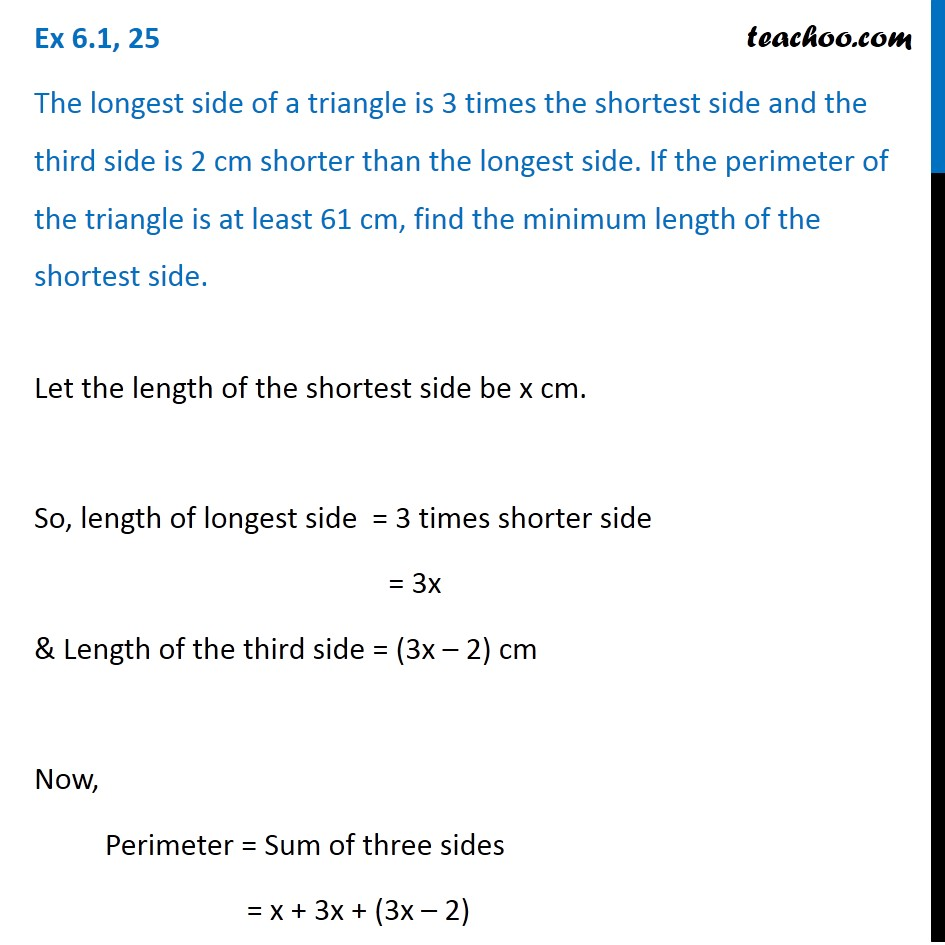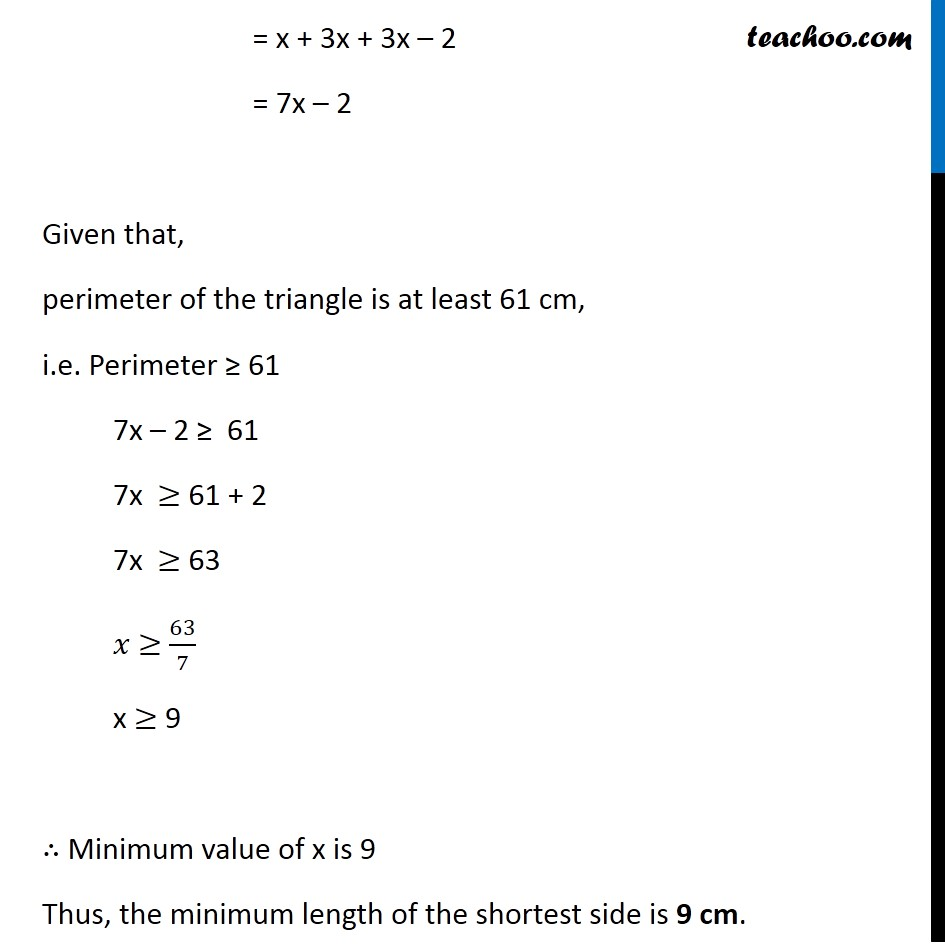Ex 5.1

Chapter 5 Class 11 Linear Inequalities
Serial order wiseLearn in your speed, with individual attention - Teachoo Maths 1-on-1 Class

### Transcript

Ex 5.1, 25 The longest side of a triangle is 3 times the shortest side and the third side is 2 cm shorter than the longest side. If the perimeter of the triangle is at least 61 cm, find the minimum length of the shortest side. Let the length of the shortest side be x cm. So, length of longest side = 3 times shorter side = 3x & Length of the third side = (3x – 2) cm Now, Perimeter = Sum of three sides = x + 3x + (3x – 2) = x + 3x + 3x – 2 = 7x – 2 Given that, perimeter of the triangle is at least 61 cm, i.e. Perimeter ≥ 61 7x – 2 ≥ 61 7x ≥ 61 + 2 7x ≥ 63 𝑥 ≥ 63/7 x ≥ 9 ∴ Minimum value of x is 9 Thus, the minimum length of the shortest side is 9 cm.